# Introduction

In a problem of location, we seek to find the optimal location of a service, or a set of them, so that the quality that the service provides to a set of demand points is, according to a given performance measure, optimal. Some examples of localization problems are:

• Find the optimal location of the central warehouse of a merchandise distribution network so that the total cost of transport is minimized
• Find the optimal location of an ambulance that must attend to the patients of a certain region so that the time in treating the farthest patient is minimized

There are numerous contexts in which localization problems arise, due to this, the location theory has been object of great attention in recent years, being able to say that it is a subject of great importance. The appearance of new facets of the problem didn’t handle until now contributes to this. For example, along with the already classic criteria for minimizing costs, new criteria appear: environmental, social, quality of life, etc. These new aspects of the problem make it an open field of study.

The package presented is devoted to solving the problem of locating a single point in the plane, using as an objective the minimization of the sum of the weighted distances to the demand points. New versions of the package will include new location models.

# The class of objects loca.p

In a planar location problem, the set of demand points is given by the coordinates of said points. Optionally, a weighting can be assigned to said points, which gives more importance to some points than to others, since the objective considered is to minimize the weighted sum of the distances between the service point and said requesting assembly. For example, if the location of a regional hospital is sought, the demand points can be the towns to which the hospital must attend and the weights of the population of each locality.

For the resolution of these problems, a class of objects designated loca.p has been defined, so that a loca.p object stores the coordinates of the demand points and the weights of each of the points. Each object loca.p has three slots, x and y that store the coordinates and w that stores the weights. When the weights are not given explicitly, all the points of demand will be considered equally important.

The rest of this section will explain how to do basic operations with loca.p objects.

## Creating class objects loca.p

Consider a location problem in which the set of demand points is $$(0,0)$$, $$(4,0)$$ and $$(2,2)$$. To create a loca.p object that represents that set, it can be done by calling the constructor function using the vector with the coordinates $$x$$ and the vector with the $$y$$ coordinates of the set of points as arguments:

loca.p(c(0, 4, 2), c(0, 0, 2))
#> An object of class "loca.p"
#> Slot "x":
#>  0 4 2
#>
#> Slot "y":
#>  0 0 2
#>
#> Slot "w":
#>  1 1 1
#>
#> Slot "label":
#>  ""

or alternatively:

loca.p(x = c(0, 4, 2), y = c(0, 0, 2))

The constructor has two more optional arguments, the third w is used to specify a vector of weights and the fourth to specify a label that will be used to identify the object. If, using the same set of points, we want to assign the weights 1, 1, 3, to said points and the label “Problem 1”, we use:

loca.p(x = c(0, 4, 2), y = c(0, 0, 2), w = c(1, 1, 3), label = "Problem 1")
#> An object of class "loca.p"
#> Slot "x":
#>  0 4 2
#>
#> Slot "y":
#>  0 0 2
#>
#> Slot "w":
#>  1 1 3
#>
#> Slot "label":
#>  "Problem 1"

A loca.p object can also be obtained by converting a data.frame object that has the x and y columns, and optionally w. Starting from data.frame

d
#>    x y w
#> 1  0 0 1
#> 2 10 0 3
#> 3  2 8 1

you can build a loca.p object by calling the as function:

as(d, "loca.p")

or alternatively:

as.loca.p(d)

Reciprocally, a loca.p object can be converted into a data.frame object by:

p1 <- loca.p(x = c(0, 4, 2), y = c(0, 0, 2), w = c(1, 1, 3), label = "Problem 1")
as(p1, 'data.frame')
#>   x y w
#> 1 0 0 1
#> 2 4 0 1
#> 3 2 2 3

or alternatively

as.data.frame(p1)

In conversions, the label slot of the loca.p object is stored as an attribute of the data.frame object. The label can be read and modified by accessing them:

p1@label
#>  "Problem 1"
dp1 <- as.data.frame(p1)
attr(dp1, "label")
#>  "Problem 1"

The loca.p objects can also be transformed into or constructed from objects of type matrix.

## Random generation of class objects loca.p

Random objects of class loca.p can be created using the rloca.p function. The first argument, n indicates the number of points to generate. By default, these points are generated in the unit square $$[0,1] \times [0, 1]$$. Thus, to generate a loca.p object with 5 points in the unit square, we use:

set.seed(161236)
rloca.p(5)
#> An object of class "loca.p"
#> Slot "x":
#>  0.12405385 0.50263749 0.15097058 0.02051109 0.57587429
#>
#> Slot "y":
#>  0.2435999 0.5788917 0.7562588 0.1144796 0.2721178
#>
#> Slot "w":
#>  1 1 1 1 1
#>
#> Slot "label":
#>  ""

The arguments xmin, xmax, ymin and ymax allow you to specify the rectangle in which the points will be generated. In addition, the rloca.p function allows you to specify the label for the new object. For example, to generate the points in the rectangle $$[- 1, 1] \times[-5,5]$$ with the label “Rectangle” is used:

rloca.p(5, xmin = -1, xmax = 1, ymin = -5, ymax = 5, label = "Rectangle")
#> An object of class "loca.p"
#> Slot "x":
#>  -0.9452062  0.5769137  0.8819392 -0.6214939 -0.1391079
#>
#> Slot "y":
#>  -3.325587  3.931197 -4.497122 -1.304617 -2.815923
#>
#> Slot "w":
#>  1 1 1 1 1
#>
#> Slot "label":
#>  "Rectangle"

The points generated by the rloca.p function can be generated in spatially distributed groups. The groups argument allows you to specify the number of groups by a number or the number of points in each group through a vector. In this second case, the value given to the n argument is ignored. To randomly generate a demand set with three equal groups:

rloca.p(9, groups = 3, label = "Three equal groups")
#> An object of class "loca.p"
#> Slot "x":
#>  1.0435201 1.1954117 1.6470744 0.7868431 0.7648655 0.8508291 0.7502594
#>  1.0788618 1.1116052
#>
#> Slot "y":
#>  1.1400092 0.6076905 0.8163944 1.3954599 1.3186699 0.9262516 0.9044921
#>  0.9464590 0.7384576
#>
#> Slot "w":
#>  1 1 1 1 1 1 1 1 1
#>
#> Slot "label":
#>  "Three equal groups"

for three unequal groups:

rloca.p(groups = c(2, 2, 5), label = "Three unequal groups")
#> An object of class "loca.p"
#> Slot "x":
#>  1.6689680 0.9978501 0.5592493 0.4330953 0.7297446 1.1752629 0.9298473
#>  0.4017964 0.8527443
#>
#> Slot "y":
#>  0.9097321 0.4319344 1.4223225 1.4137017 0.5544933 1.2903588 0.4965480
#>  1.0362726 0.4947184
#>
#> Slot "w":
#>  1 1 1 1 1 1 1 1 1
#>
#> Slot "label":
#>  "Three unequal groups"

To generate the data in groups, an offset of the center of each group is generated first and then the points are generated by adding to each point the offset that corresponds to their group. For this reason, groups = 1 is not equivalent to not specifying that parameter. The offset of the centers can be specified by the arguments xgmin, xgmax, ygmin and ygmax. To illustrate better how the function works, the result can be painted:

rl <- rloca.p(60, groups = 3, xmin = -10, xmax = 10, ymin = -10, ymax = 10, xgmin = -1, xgmax = 1, ygmin = -1, ygmax = 1, label = "Three groups")
plot(rl)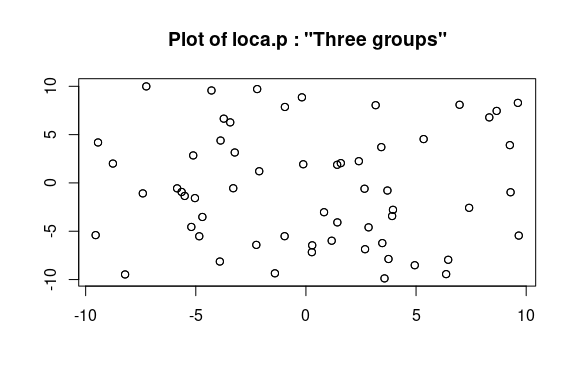## Summaring up the data

To obtain a numeric summary of a loca.p object you can use the summary function:

summary(rl)
#>         label  n      xmin    xwmean     xmax      ymin     ywmean     ymax
#>  Three groups 60 -9.546496 0.1722399 9.662573 -9.882184 -0.5505148 9.990476

The summary shows the minimum, maximum and average values of both coordinates, in addition to the weighted averages of the coordinates of the points for each component.

# Weighted average distance

Given a loca.p object, we can evaluate the weighted distance from a given point. Likewise, the gradient of said function can be evaluated and the problem of minimizing said objective can be solved.

## Evaluation

The weighted average distance function is called distsum in the package, given a point, for example: $$(3, 1)$$ you can evaluate the weighted average distance to a loca.p object:

pt3 <- loca.p(x = c(0, 4, 2), y = c(0, 0, 2), label = "Three points")
distsum(o = pt3, x = 3, y = 1)
#>  5.990705

You can also calculate the gradient of distsum called distsumgra:

distsumgra(o = pt3, x = 3, y = 1)
#>  0.9486833 0.3162278

## Resolution

To find the optimal solution to the previous location problem, use the distsummin function:

s <- distsummin(pt3)
s
#>  2.00000 1.15332

Evaluating the function and the gradient at the point obtained

distsum(o = pt3, x = s, y = s)
#>  5.464102
distsumgra(o = pt3, x = s, y = s)
#>   3.110246e-07 -8.970172e-04

As can be verified by the value of the gradient, the solution found is a local optimum and, since the convex objective function, is a global optimum.

These three functions support an optional lp argument, if this argument is omitted, the Euclidean standard is used, that is, the $$l_2$$ rule, if a value is specified for lp the $$l_p$$ rule will be used for that value of $$p$$.

Note that specifying lp = 2 uses the generic algorithm for the l_p rule with $$p$$ equal to 2. The use of the generic algorithm requires a greater computational effort to solve the problem, so it is not advisable to specify this argument to use the Euclidean norm.

# Plotting

Both the loca.p objects and the objective function can be plotted in a graph. For the objective function a representation based on level curves and another on a 3D graph is provided.

## Plot an object loca.p

The graph of a loca.p object consists of plotting the scatter plot of the set of demand points in the plane using the plot function:

plot(pt3)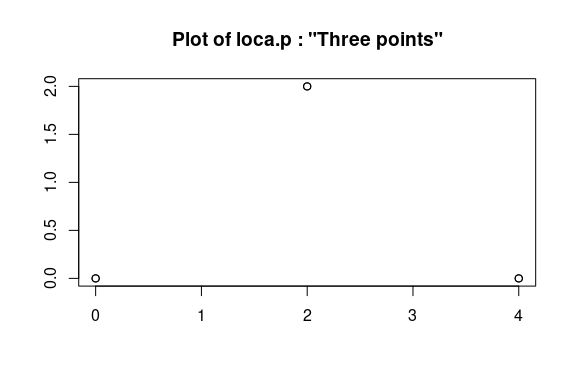## Contour graph

The contour graph is made with the contour function:

contour(pt3)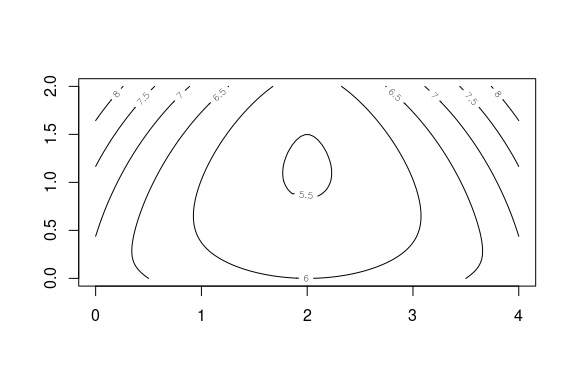In the graph you can see how the function reaches the minimum at the point previously calculated. Expanding:

contour(pt3, xlim = c(1.9, 2.1), ylim = c(1, 1.2), levels = c(5.465, 5.47, 5.475))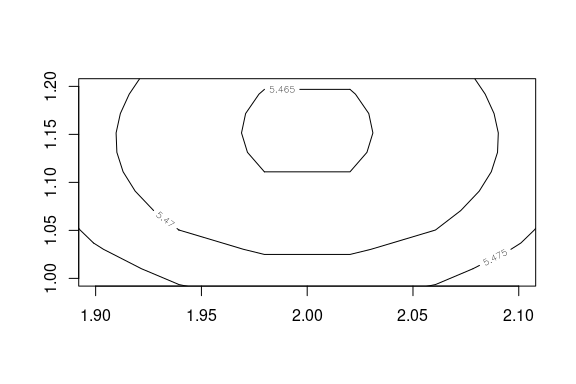The plot and contour functions support an optional img argument that allows you to specify a raster graphic to be used as the background of the graphic.

## 3D graph

Analogously, a three-dimensional representation can be made using the persp function:

persp(pt3)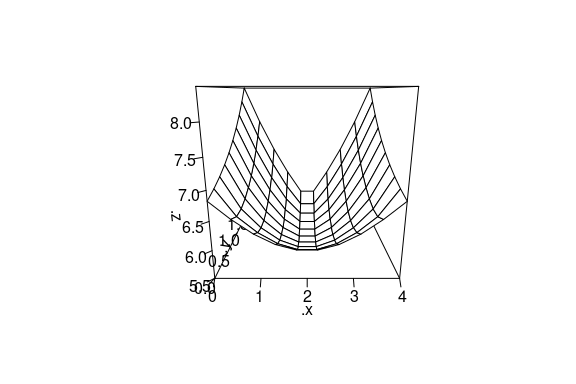persp(pt3, col = "lightblue", theta = 45, ltheta = 120, shade = 0.75, ticktype = "detailed")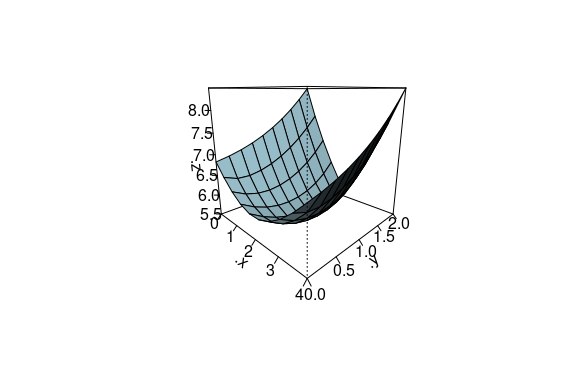The three representation functions will pass the remaining optional arguments to the generic function plot.

## Example of location in Andalusia

The data of the Andalusian capitals are loaded and it becomes a class object loca.p:

data(andalusia)
o <- loca.p(x = andalusia$x[1: 8], y = andalusia$y[1: 8])

The bounds values for the graph are calculated:

xmin <- min(andalusia$x) ymin <- min(andalusia$y)
xmax <- max(andalusia$x) ymax <- max(andalusia$y)

The map of Andalusia is loaded and the points are represented with the background map

file = system.file('img', 'andalusian_provinces.png', package = 'orloca')
plot(o, img = img, main = 'Andalusia', xleft = xmin, ybottom = ymin, xright = xmax, ytop = ymax)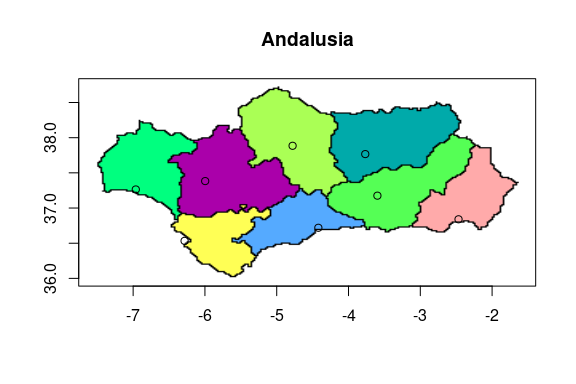The contour graph is:

contour(o, img = img, main = 'Andalusia', xleft = xmin, ybottom = ymin, xright = xmax, ytop = ymax)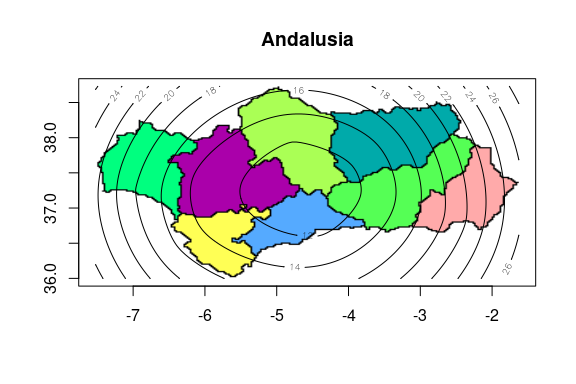The optimal solution of the location problem with the 8 capitals, eight first rows, is obtained:

andalusia.loca.p <- loca.p(andalusia$x[1: 8], andalusia$y[1: 8])
sol <- distsummin(andalusia.loca.p)
sol
#>  -4.610679 37.248691

The optimal solution provided by the algorithm is located about 35 km north of Antequera. Recall that Antequera is usually considered the geographic center of Andalusia. The graph presents the solution as a red dot:

contour(o, img = img, main = 'Andalusia', xleft = xmin, ybottom = ymin, xright = xmax, ytop = ymax)
points(sol, sol, type = 'p', col = 'red')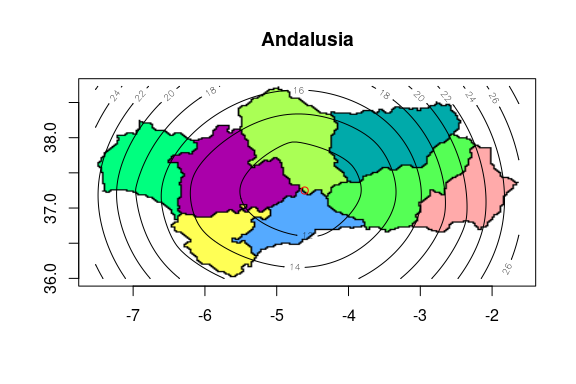For simplicity in the example, the terrestrial curvature has not been taken into account.